# HSPT Math : Algebra

## Example Questions

← Previous 1 3 4 5 6 7 8 9 57 58

### Example Question #1 : Algebra

If you rewrite the phrase "the product of nine and a number added to the sum of six and twice the number" as an algebraic expression, then simplify the expression, the result is: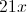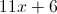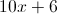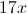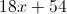Explanation:

"The product of nine and a number" is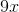.  "Twice the number" is, and "The sum of six and twice the number" is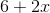"The product...added to the sum..." is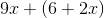; simplify to get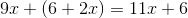### Example Question #1 : Algebra

Simplify the expression: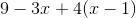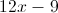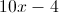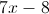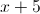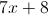Explanation: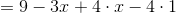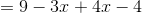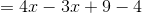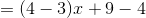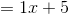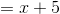### Example Question #1 : Algebra

Simplify: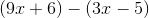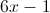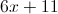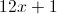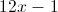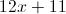Explanation: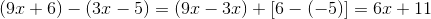### Example Question #21 : Operations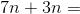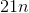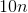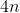Explanation:

Add the numbers and keep the variable: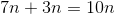Answer:### Example Question #1 : Algebra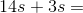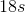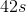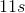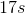Explanation:

Add the numbers and keep the variable: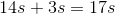Answer:### Example Question #22 : Variables

Simplify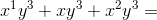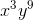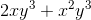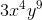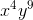Explanation:

In order to add variables the terms must be like. In order for terms to be like, the variables must be exactly alike also being raised to the same power by the exponent.

In this case the like terms are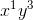and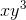. Just because there is a 1 in the exponent for the first term doesnt mean it is different from the second term. With exponents if a variable does not show an exponent, that means it is still to the first power.

We add the coefficients of the like terms. The coefficient is the number in front of the first variable, in this case it is 1 for both terms because of the identity property of multiplication stating any variable, term, or number multiplied by 1 is itself.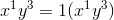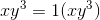Our last term is not like because thevariable is raised to a different power than the other two. In this case we do not combine it to the like terms, we just add it to the end of the term.

### Example Question #151 : Operations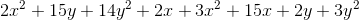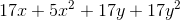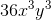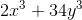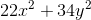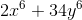Explanation:

Remember, for exponent problems, you group together different exponents and different combinations of variables as though each were a different type of variable.  Therefore, you can group your problem as follows: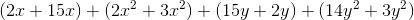Now, just combine like terms:### Example Question #2262 : Isee Middle Level (Grades 7 8) Mathematics Achievement

Simplify: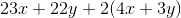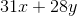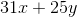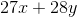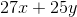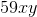Explanation:

You should begin by distributingthrough the whole group that it precedes: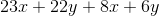Now, move your like variables next to each other: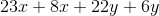Finally, combine the like terms:### Example Question #1 : Algebra

Simplify: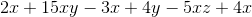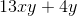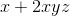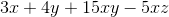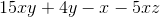Explanation:

First, group together your like variables: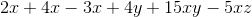The only like variables needing to be combined are the x-variables.  You can do this in steps or all at once: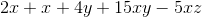### Example Question #1 : Algebra

Simplify: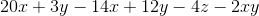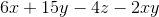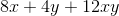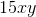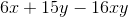Explanation:

First, move the like terms to be next to each other: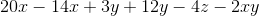Now, combine the x-variables and the y-variables:← Previous 1 3 4 5 6 7 8 9 57 58

### All HSPT Math Resources